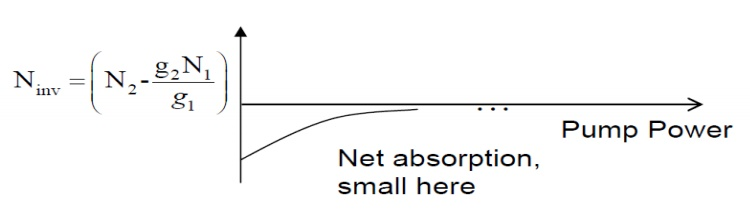# Level Lasers

1. Two-Level Laser 2. Three Level Laser 3. Quasi Three Level Laser

Level Lasers

Every atom or molecule in nature has a specific structure for its energy levels. The lowest energy level is called the ground state, which is the naturally preferred energy state. As long as no energy is added to the atom, the electron will remain in the ground state. When the atom receives energy (electrical energy, optical energy, or any form of energy), this energy is transferred to the electron, and raises it to a higher energy level (in our model further away from the nucleus).The atom is then considered to be in an excited state. The electron can stay only at the specific energy states (levels) which are unique for each specific atom. The electron cannot be in between these "allowed energy states", but it can "jump" from one energy level to another, while receiving or emitting specific amounts of energy.

These specific amounts of energy are equal to the difference between energy levels within the atom. Each amount of energy is called a "Quantum" of energy (The name "Quantum Theory" comes from these discrete amounts of energy). Energy transfer to and from the atom Energy transfer to and from the atom can be performed in two different ways:

1. Two-Level LaserSuppose we try to increase N2 with strong light at hν to create a population inversion2. Three Level LaserIn a three level system, the terminal level for the fluorescence process is the ground level(ie) the level with the lowest energy. Here, The population inversion is produced by raising electrons to the high energy level by the process of pumping with an auxiliary light source. It is observed to excite electrons from level 1 to level 3. Then , a very fast radiation less transition accomplished by thermal vibrations of the atoms will drop the electrons to level 2. The difference in energy between levels 3 and 2 appears as heat. Stimulated emission occurs between levels 2 and 1 at frequency,

It substantial power at frequency f3is supplied, the transition rate from level 1 to 3 will be large.

3. Quasi Three Level Laser

1.Collisions with other atoms, and the transfer of kinetic energy as a result of the collision. This kinetic energy is transferred into internal energy of the atom.2. Absorption and emission of electromagnetic radiation. Since we are now interested in the lasing process, we shall concentrate on the second mechanism of energy transfer to and from the atom (The first excitation mechanism is used in certain lasers, like Helium-Neon, as a way to put energy into the laser.

The interactions between electromagnetic radiation and matter cause changes in the energy states of the electrons in matter.

•Electrons can be transferred from one energy level to another, while absorbing or emitting a certain amount of energy. This amount of energy is equal to the energy difference between these

two energy levels (E2-E1).

•When this energy is absorbed or emitted in a form of electromagnetic radiation, the energy difference between these two energy levels (E2-E1) determines uniquely the frequency (ν) of theelectromagnetic radiation: ( ∆E) = E2-E1= hν= h(bar)ω

Eg:The laser is a system that is similar to an electronic oscillator. An Oscillator is a system that produces oscillation s without an external driving mechanism.

Study Material, Lecturing Notes, Assignment, Reference, Wiki description explanation, brief detail
Fiber optics and Laser instruments : Laser Fundamentals : Level Lasers |